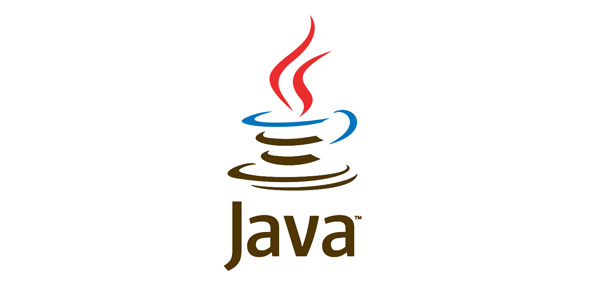# Scjp 6.0 _ Java _ Level 1

10 Questions | Total Attempts: 316Settings• 1.
Given: public class TestString1 {     public static void main(String[] args) {         String str = "420";         str += 42;         System.out.print(str);     } } What is the output?
• A.

42

• B.

420

• C.

462

• D.

42042

• E.

Compilation fails.

• F.

An exception is thrown at runtime.

• 2.
Given: 35. String #name = "Jane Doe"; 36. int \$age = 24; 37. Double _height = 123.5; 38. double ~temp = 37.5;  Which two statements are true? (Choose two.)
• A.

Line 35 will not compile.

• B.

Line 36 will not compile.

• C.

Line 37 will not compile.

• D.

Line 38 will not compile.

• 3.
Given: public class Test {     public static void main(String[] args) {         int x = 5;         boolean b1 = true;         boolean b2 = false;         if ((x == 4) && !b2)             System.out.print("1 ");             System.out.print("2 ");         if ((b2 = true) && b1)             System.out.print("3 ");     } } What is the result?
• A.

2

• B.

3

• C.

12

• D.

23

• E.

123

• F.

Compilation fails.

• G.

An exception is thrown at runtime.

• 4.
Given:     public void go() {         String o = "";         z:         for (int x = 0; x < 3; x++) {             for (int y = 0; y < 2; y++) {                 if (x == 1)break;                 if (x == 2 && y == 1)break z;                 o = o + x + y;             }         }         System.out.println(o);     } What is the result when the go() method is invoked?
• A.

00

• B.

0001

• C.

000120

• D.

00012021

• E.

Compilation fails.

• F.

An exception is thrown at runtime.

• 5.
Given:     int x = 0;     int y = 10;     while (x < 5) {         y--;         ++x;     } ;     System.out.print(x + "," + y); What is the result?
• A.

5,6

• B.

5,5

• C.

6,5

• D.

6,6

• 6.
Given: public class Breaker2 {     static String o = "";     public static void main(String[] args) {         z: for (int x = 2; x < 7; x++) {             if (x == 3)                 continue;             if (x == 5)                 break z;             o = o + x;         }         System.out.println(o);     } } What is the result?
• A.

2

• B.

24

• C.

234

• D.

246

• E.

2346

• F.

Compilation fails.

• 7.
Given:     String[] elements = {"for", "tea", "too"};     String first = (elements.length>0) ? elements : null; What is the result?
• A.

Compilation fails.

• B.

An exception is thrown at runtime.

• C.

The variable first is set to null.

• D.

The variable first is set to elements.

• 8.
Given: class Alligator {     public static void main(String[] args) {         int[] x[] = { { 1, 2 }, { 3, 4, 5 }, { 6, 7, 8, 9 } };         int y[][] = x;         System.out.print(y);     } } What is the result?
• A.

2

• B.

3

• C.

4

• D.

6

• E.

7

• F.

Compilation fails.

• 9.
Given: public class Barn {     public static void main(String[] args) {         new Barn.go("hi", 1);         new Barn.go("hi", "world", 2);     }     public void go(String... y, int x) {         System.out.print(y[y.length - 1] + " ");     } } What is the result?
• A.

Hi hi

• B.

Hi world

• C.

World world

• D.

Compilation fails.

• E.

An exception is thrown at runtime.

• 10.
Given: 1. class Super{ 2.     private int a; 3.     protected Super(int a){this.a = a;} 4. } ... 11. class Sub extends Super{ 12.     public Sub(int a){super(a);} 13.     public Sub(){this.a = 5;} 14. } Which two, independently, will allow Sub to compile? (Choose two.)
• A.

Change line 2 to: 　public int a;

• B.

Change line 2 to: 　protected int a;

• C.

Change line 13 to: 　public Sub(){this(5);}

• D.

Change line 13 to: 　public Sub(){super(5);}

• E.

Change line 13 to: 　public Sub(){super(a);}

Related TopicsBack to top